# Ancient China Mathematics

Ancient Chinese developed mathematics independently of other cultures. By the 11th century BCE, they had algebra, geometry, trigonometry, decimals, negative numbers, and a binary system. In the 13th century, a Chinese mathematician named Zhu Shijie developed the method of four unknowns.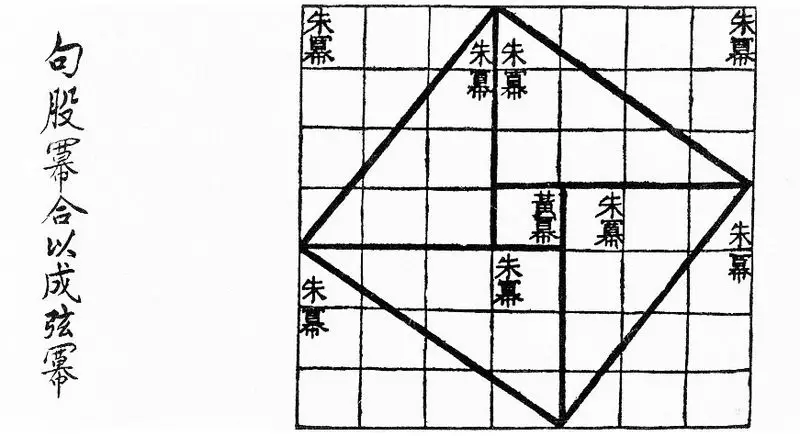Two important books on mathematics called The Nine Chapters on the Mathematical Art, and the Writings on Reckoning were written during the Han period.

Both books gave instructions on how to solve mathematical problems in daily. Both texts comprised the use of fractions and negative numbers.

Chinese mathematics – as well as the resto of Chinese culture – was not under the influence of the ancient Mediterranean world. It was too far away, and the languages were too different.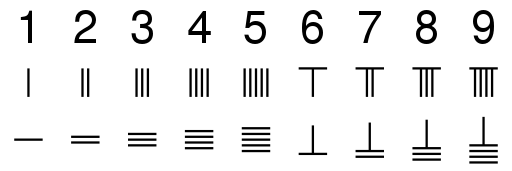While they hadn’t known about Pythagoras, they made the theorem that is the same as the Pythagorean in the Duke of Zhou’s time. Also, what we now know as Pascal’s triangle existed in China long before Pascal.

### Early Chinese mathematics

• I Ching (Yi Jing) is one of the oldest mathematical works that survived past ancient time. It contained binary numbers and hexagrams.
• During the Shang Dynasty (1600–1050 BC), Chinese used simple mathematics, which was recorded on the Oracle bone script.
• During the Zhou Dynasty, all students were required to learn math as it was one of the six crucial fields in Chinese education, called the Six Arts.
• Around 330 BCE, the philosophical Mohist canon, or Mo Jing, included substantial work on geometry. It offered one of the oldest definitions of the geometrical point, parallels, the comparison of lengths, and principles of space and bounded space.
• A text called the Zhoubi Suanjing offers a comprehensive proof of the Gougu Theorem, which is a special case of the Pythagorean Theorem that focuses on astronomical calculations.
• The first known decimal multiplication table appeared around 305 BCE.
• The abacus and “calculation with rods” were first mentioned in the second century BCE.
• There are no surviving records on the Qin dynasty mathematics, but it was certainly on an advanced level. The knowledge of geometry and precise calculations of proportions were necessary for the construction of buildings, temples, life-sized statues, and the Great Wall of China.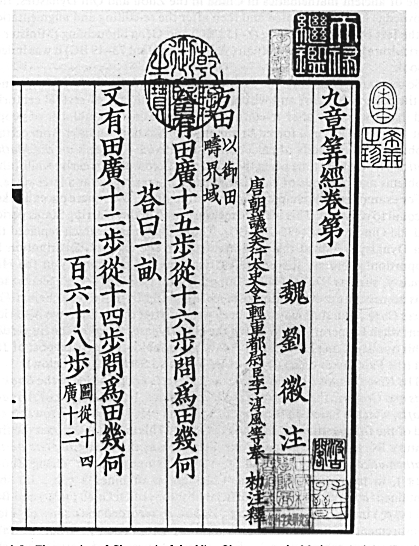### The Han Dynasty Mathematics

• The Han Chinese had a place value decimal system, and for basic computations, they used chousuan, which was a kind of counting board with rods. They knew about negative numbers, and they performed addition, subtraction, multiplication, and division.
• The Nine Chapters on the Mathematical Art and the Writings on Reckoning (also known as the Books of Computations) were produced during the Han period in ancient Chinese history. Those texts inform us how the Chinese used math in daily life – for distribution of land or division of payment.

### Writings on Reckoning

• Also known as the Book of Computations (in Chinese: Suàn shù shū), this text consists of several short independent units that address basic problems, including the use of an “excess and deficiency” method and a way to solve systems of two equations with two unknowns.

### The Nine Chapters on the Mathematical Art

• This is the most important ancient Chinese book on mathematics. It consists of 264 mathematical problems – related to surveying, taxation, architecture, agriculture, and other fields – followed by detailed solving procedure and answers.
• The Nine Chaptersoffered further improvement of linear algebra, solving systems of linear equations with two unknowns – and even solving four equations with five unknowns.
• This text included explanations of methods similar to Gaussian elimination and back substitution.
• The Nine Chapterson the Mathematical Art later became a part of the key work of mathematical education in later centuries, called The Ten Computational Canons.

### Q&A

Did ancient Greek mathematicians, such as Pythagoras, influence the development of Chinese mathematic?

No, ancient Chinese math developed independently.

What is I Ching (Yi Jing)?

It is one of the oldest mathematical works that survived past ancient time

What was the name of the work on geometry that offered early definitions of the geometrical point, parallels, the comparison of lengths, and other geometry terms?

It was Mo Jing.

Did ancient Chinese use abacus?

Yes, it has been recorded that they used an abacus in the second century BCE.

What are the two key texts on mathematics from the Han period?

Those were the Nine Chapters on the Mathematical Art and the Writings on Reckoning.

•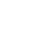History
•Science
•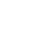Geography
•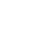Biography
•US History